# The hedging hyperprior for the normal distribution: unknown mean, known precision

## 2023-02-28

suppressMessages(suppressWarnings(library(MASS)))
suppressMessages(suppressWarnings(library(tidyverse)))
knitr::opts_chunk$set(echo=TRUE, fig.width=8, fig.height=2) I want to write a paper about the hedging hyperprior. I need to work out some simple examples first. Here is an example involving the normal distribution. ### A simple Bayesian analysis with an informative prior. There are lots of guides on the Internet on using a normal prior for data that is normally distributed with the population standard deviation $$\sigma$$ known or unknown. I used papers written by Kevin P. Murphy and Michael I. Jordan to get these formulas. For simplicity, all sums and products are assumed to go from i=1 to n. Consider a Bayesian analysis of an outcome variable that has a normal distribution. The density of the normal distribution is $$f(X; \mu, \sigma) = \frac{1}{\sigma \sqrt{2 \pi}}e^{-(X-\mu)^2 / 2\sigma^2}$$ For this derivation, it is easier to write the density in terms of $$f(X; \mu, \tau) = \sqrt{\frac{\tau}{2 \pi}}e^{-\frac{1}{2}\tau (X-\mu)^2}$$ where $$\tau=\frac{1}{\sigma^2}$$ The parameter $$\tau$$ is called the precision. Note that some define the precision differently as the inverse of the standard deviation rather than the inverse of the variance. Assume that $$\tau$$ is known and equal to $$\tau_F$$. Other cases with mean known and precision unknown and with both mean and precision unknown appear on different web pages. For simplicity, we will drop any multiplicative constants not involving X or $$\mu$$ $$f(X; \mu) \propto e^{-\frac{1}{2}\tau_F(X-\mu)^2}$$ The conjugate prior for a normal distribution is also a normal distribution. $$g(\mu; \mu_0, \tau_0) \propto e^{- \frac{1}{2}\tau_0(\mu-\mu_0)^2}$$ where the prior mean is $$\mu_0$$ and the prior precision is $$\tau_0$$. For an informative prior distribution, $$\tau_0$$ is usually much smaller than $$\tau_F$$. The ratio $$n_0=\tau_F/\tau_0$$ is the effective prior sample size and is equivalent to adding $$n_0$$ pseudo data values of $$\mu_0$$ to the observed data. You have collected a sample of n data points, $$\mathbf{X} = X_1, X_2, ..., X_n$$. This gives you a likelihood of $$L(\mathbf{X} | \mu) \propto e^{- \frac{1}{2} \tau_F\sum(X_i-\mu)^2}$$ Rewrite this by incorporating $$\bar{X}$$ into the squared term $$L(\mathbf{X} | \mu) \propto e^{-\frac{1}{2} \tau_F \sum (X_i-\bar{X}+\bar{X}-\mu)^2}$$ When you square the term inside the sum, the cross-product cancels out, leaving you with $$L(\mathbf{X} | \mu) \propto e^{- \frac{1}{2} \tau_F (\sum(X_i-\bar{X})^2} e^{- \frac{n}{2} \tau_F (\bar{X}-\mu)^2}$$ $$L(\mathbf{X} | \mu) \propto e^{-\frac{n-1}{2}\tau_F S^2} e^{-\frac{n}{2} \tau_F (\mu-\bar{X})^2}$$ The posterior distribution is proportional to the product of the prior and the likelihood. $$h(\mu | \mathbf{X}) \propto e^{- \frac{1}{2} \tau_0 (\mu-\mu_0)^2} e^{- \frac{n-1}{2} \tau_F S^2} e^{-\frac{n}{2} \tau_F(\mu-\bar{X})^2)}$$ Drop the middle exponential term, which is constant with respect to $$\mu$$ and use high school algebra (completing the square) to get $$h(\mu | \mathbf{X}) \propto e^{ -(n\tau_F+\tau_0) (\mu- \frac {n\tau_F\bar{X}+\tau_0\mu_0} {n\tau_F+\tau_0})^2}$$ which is a normal distribution. The posterior mean $$\frac{n \tau_F \bar{X} + \tau_0 \mu_0}{n\tau_F+\tau_0}$$ and the posterior precision is $$n \tau_F + \tau_0$$ The posterior mean can be written as a weighted average of the prior mean and the mean of the observed data. $$\frac{n \tau_F}{n\tau_F+\tau_0}\bar{X} + \frac{\tau_0}{n\tau_F+\tau_0}\mu_0$$ ### A simple numerical example x_max <- 8 mu0 <- 4 tau0 <- 8 tauF <- 0.6 n <- 10 xbar <- 2 mu1 <- (tau0*mu0+n*tauF*xbar)/(tau0+n*tauF) tau1 <- tau0+n*tauF x_grid <- seq(0.01, x_max, length=1000) f <- function(x, mu, tau) { sqrt(tau/2*pi)*exp(-0.5*tau*(x-mu)^2) } g <- f prior05 <- qnorm(0.025, mu0, 1/sqrt(tau0)) prior95 <- qnorm(0.975, mu0, 1/sqrt(tau0)) post05 <- qnorm(0.025, mu1, 1/sqrt(tau1)) post95 <- qnorm(0.975, mu1, 1/sqrt(tau1))  Let’s illustrate this with an informative prior with $$\mu_0$$ = 4 and $$\tau_0$$ = 8. g_grid <- g(x_grid, mu0, tau0) outside <- x_grid < prior05 | x_grid > prior95 x_color <- ifelse(outside, "white", "gray") data.frame(x=x_grid, y=g_grid) %>% ggplot(aes(x, y)) + geom_segment(aes(xend=x, yend=0), color=x_color) + geom_line() + xlab(" ") + ylab(" ")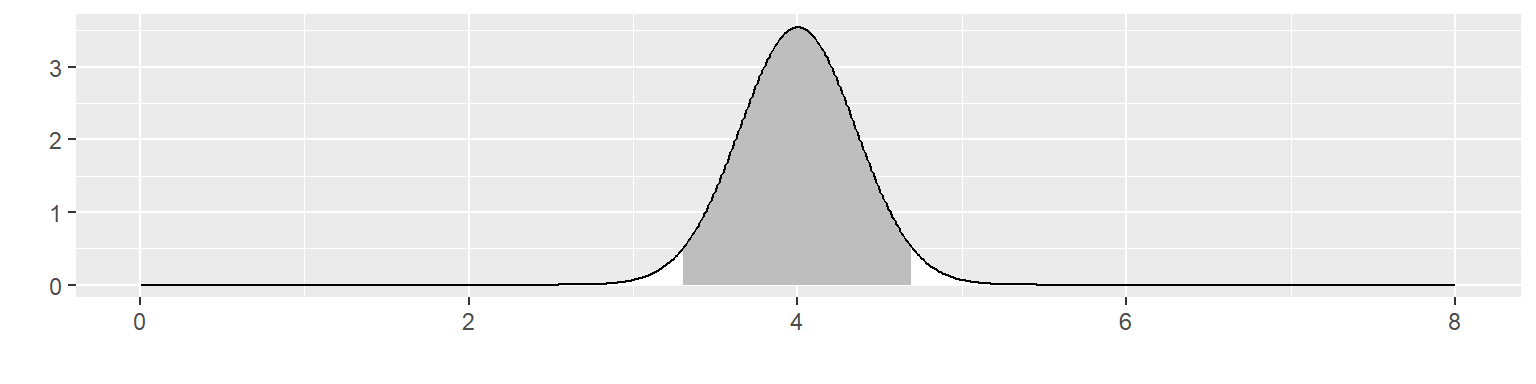This corresponds to a fairly informative prior distribution. This distribution places $$\mu$$ within 3.31 and 4.69 with 95% probability. Let’s see what happens with this informative prior if you observe 10 values of x with a mean of 2. The likelihood is f_grid <- f(xbar, x_grid, tauF)^n x_color <- "white" data.frame(x=x_grid, y=f_grid) %>% ggplot(aes(x, y)) + geom_line() + xlab(" ") + ylab(" ")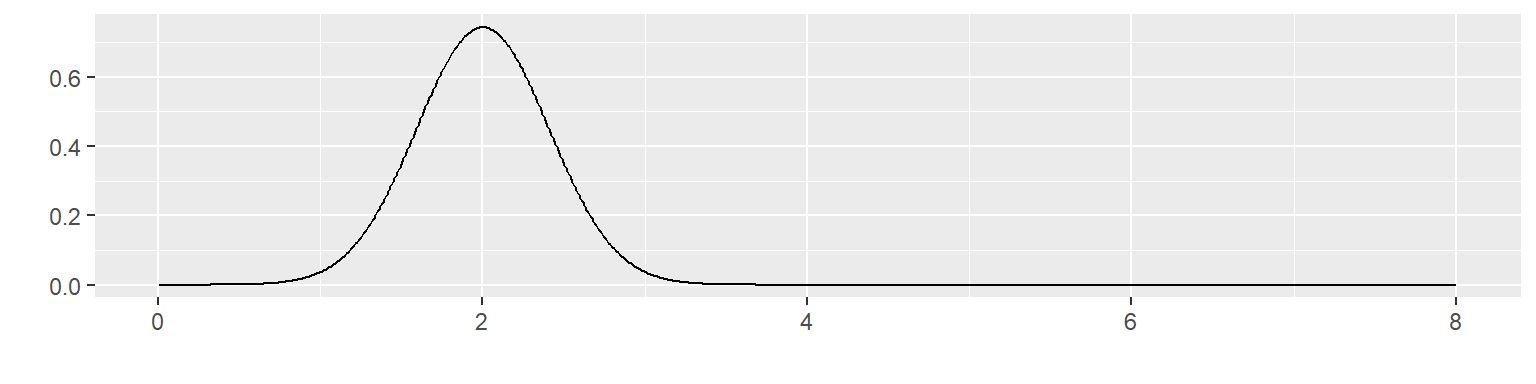Multiply these two together to get the posterior distribution. h_grid <- g(x_grid, mu1, tau1) outside <- x_grid < post05 | x_grid > post95 x_color <- ifelse(outside, "white", "gray") data.frame(x=x_grid, y=h_grid) %>% ggplot(aes(x, y)) + geom_segment(aes(xend=x_grid, yend=0), color=x_color) + geom_line() + xlab(" ") + ylab(" ")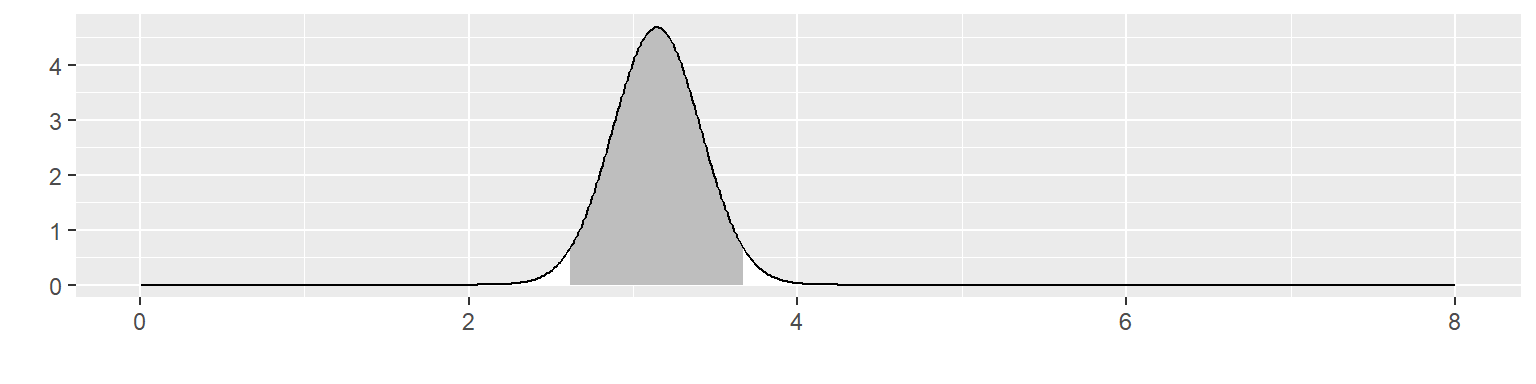The posterior distribution is not close to either the prior distribution or the likelihood. It places $$\mu$$ within 2.62 and 3.67 with 95% probability. It excludes the prior mean of 4 and it excludes 2, the mean of the data. ### The hedging hyperprior When the likelihood of your data and your informative prior are in conflict, you may wish to downweight the informative prior. You can do this by including a hedging hyperprior. Define your prior distribution as a normal distributions with prior mean $$\mu0$$ and prior precision $$\tau_F+\lambda (\tau_0-\tau_F)$$ where $$\lambda$$ ranges between 0 and 1 and $$\tau_F$$ is less than $$\tau_0$$. When $$\lambda=0$$, the prior precision reduces to $$\tau_F$$ which is equivalent to a prior sample size of $$n_0=1$$. When $$lambda=1$$, the prior precision is $$\tau_0$$ which represents the full strength of the informative priori distribution. Place a hyperprior on the parameter $$\lambda$$. The uniform(0,1) distribution is a reasonable choice for the hyperprior, but others are possible. knitr::opts_chunk$set(echo=TRUE, fig.width=8, fig.height=8)
lambda_grid <- seq(0, 1, length=60)
mu_grid <- seq(0, x_max, length=60)

gstar <- function(mu, lambda) {g(mu, mu0, lambda*(tau0-tauF)+tauF)}
density <- outer(mu_grid, lambda_grid, gstar)
op <- par(bg = "white")
z_max <- max(density, na.rm=TRUE)
persp(
mu_grid,
lambda_grid,
density,
zlim=c(0, 1.1*z_max),
xlab="mu",
ylab="lambda",
mu = 135,
phi = 30,
expand=0.5,
ticktype="detailed")
## Warning in persp.default(mu_grid, lambda_grid, density, zlim = c(0, 1.1 * : "mu"
## is not a graphical parameter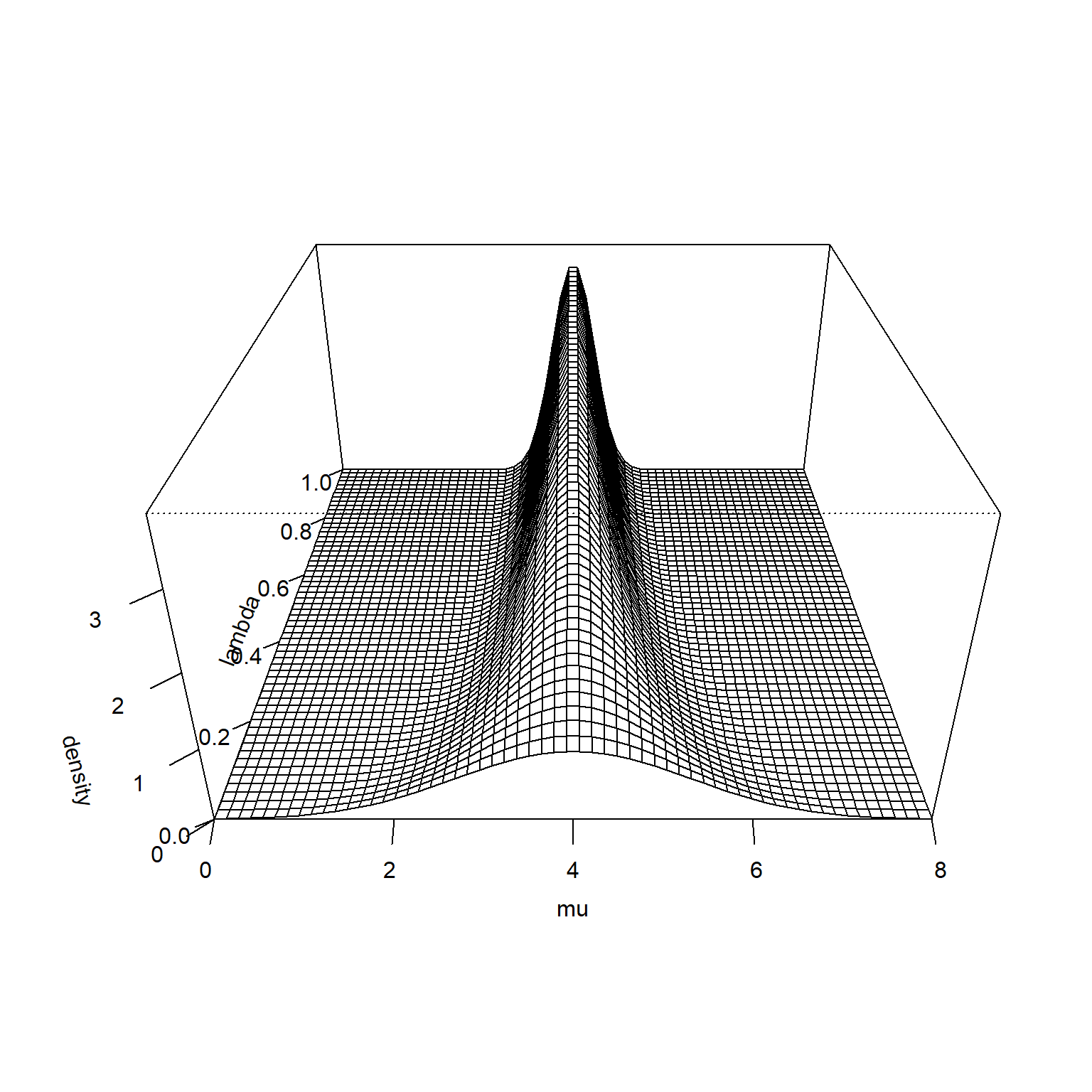This is the joint prior distribution of $$\mu_0$$ and $$\lambda$$.

It is hard to see at this scale, but the prior distribution is higher for small values of $$\lambda$$ when $$\mu$$ is very large or very small. This becomes more obvious if you remove the middle of the surface and rescale the Z-axis.

density <- outer(mu_grid, lambda_grid, gstar)
density[16:44, ] <- NA
op <- par(bg = "white")
z_max <- max(density, na.rm=TRUE)
persp(
mu_grid,
lambda_grid,
density,
zlim=c(0, 1.1*z_max),
xlab="mu",
ylab="lambda",
mu = 135,
phi = 30,
expand=0.5,
ticktype="detailed")
## Warning in persp.default(mu_grid, lambda_grid, density, zlim = c(0, 1.1 * : "mu"
## is not a graphical parameter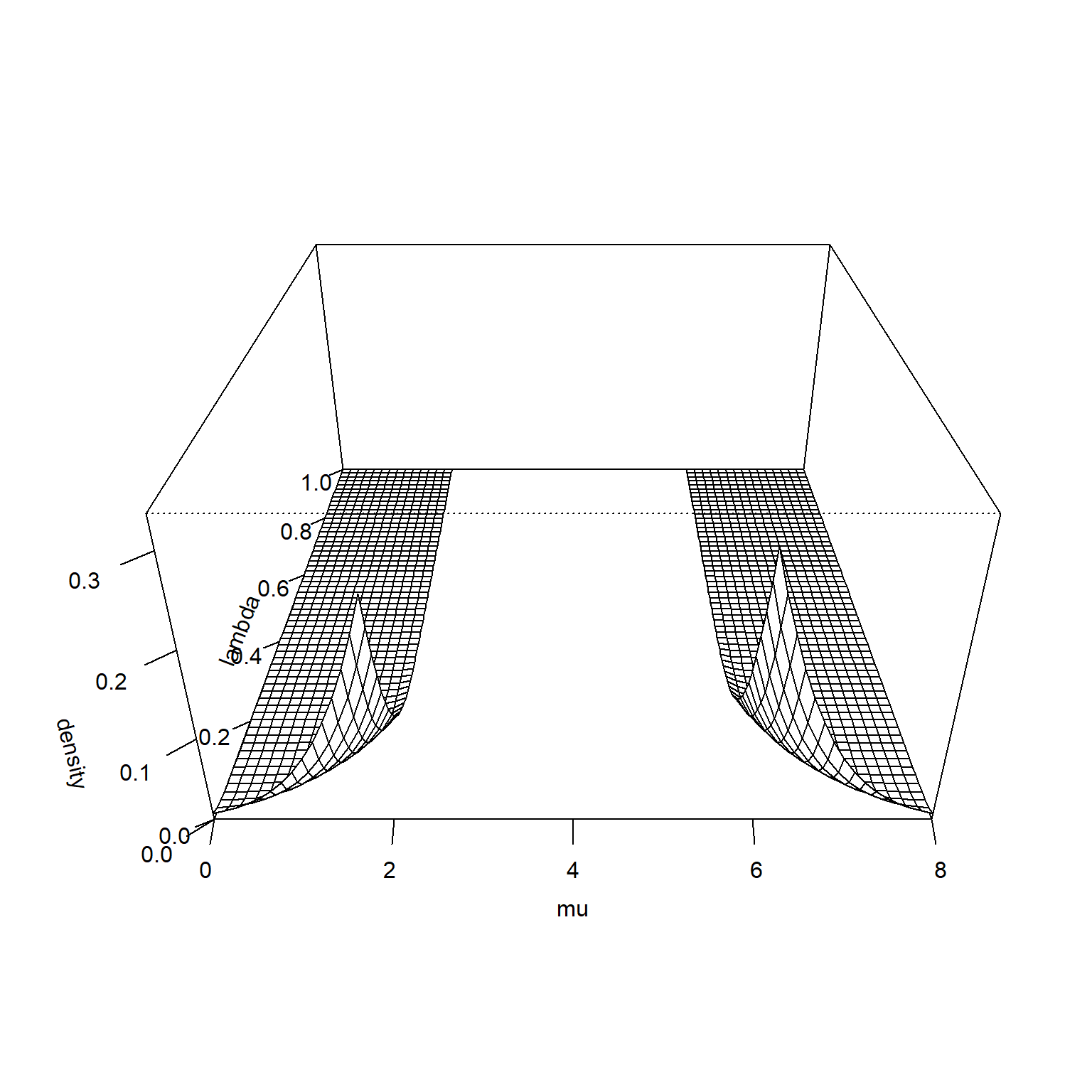The likelihood function is constant across all values of $$\tau$$, so its surface looks like the following.

lambda_grid <- seq(0, 1, length=60)
mu_grid <- seq(0, x_max, length=60)

fstar <- function(mu, lambda) {f(xbar, mu, tau0)^n}
likelihood <- outer(mu_grid, lambda_grid, fstar)
z_max <- max(likelihood, na.rm=TRUE)
persp(
mu_grid,
lambda_grid,
likelihood,
zlim=c(0, 1.1*z_max),
xlab="mu",
ylab="lambda",
mu = 135,
phi = 30,
expand=0.5,
ticktype="detailed")
## Warning in persp.default(mu_grid, lambda_grid, likelihood, zlim = c(0, 1.1 * :
## "mu" is not a graphical parameter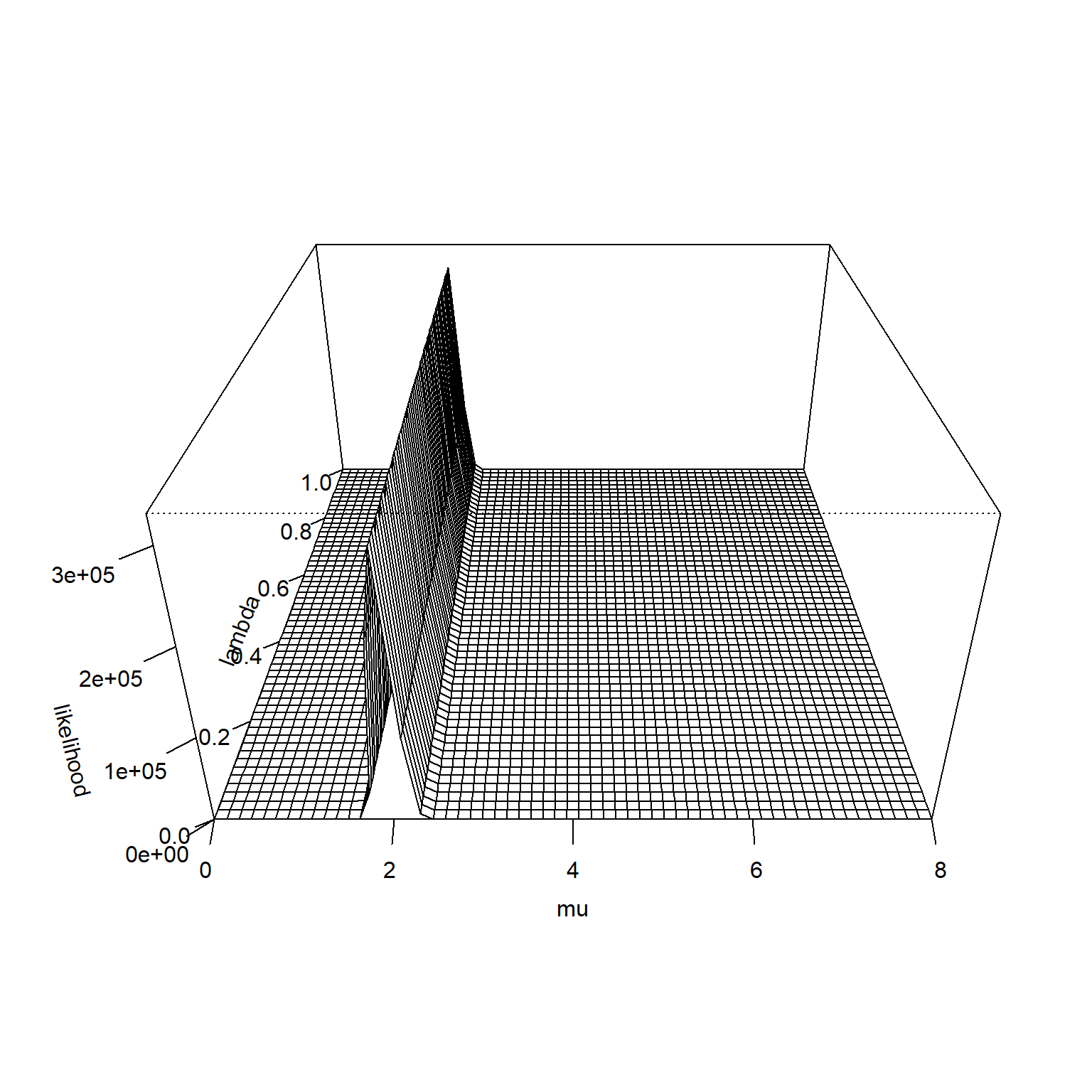The product of the prior and the likelihood is proportional to the posterior distribution.

lambda_grid <- seq(0, 1, length=60)
mu_grid <- seq(0, x_max, length=60)

hstar <- function(mu, lambda) {g(mu, mu0, lambda*(tau0-tauF) + tauF)*f(xbar, mu, tauF)^n}
density <- outer(mu_grid, lambda_grid, hstar)
z_max <- max(density, na.rm=TRUE)
persp(
mu_grid,
lambda_grid,
density,
zlim=c(0, 1.1*z_max),
xlab="mu",
ylab="lambda",
mu = 135,
phi = 30,
expand=0.5,
ticktype="detailed")
## Warning in persp.default(mu_grid, lambda_grid, density, zlim = c(0, 1.1 * : "mu"
## is not a graphical parameter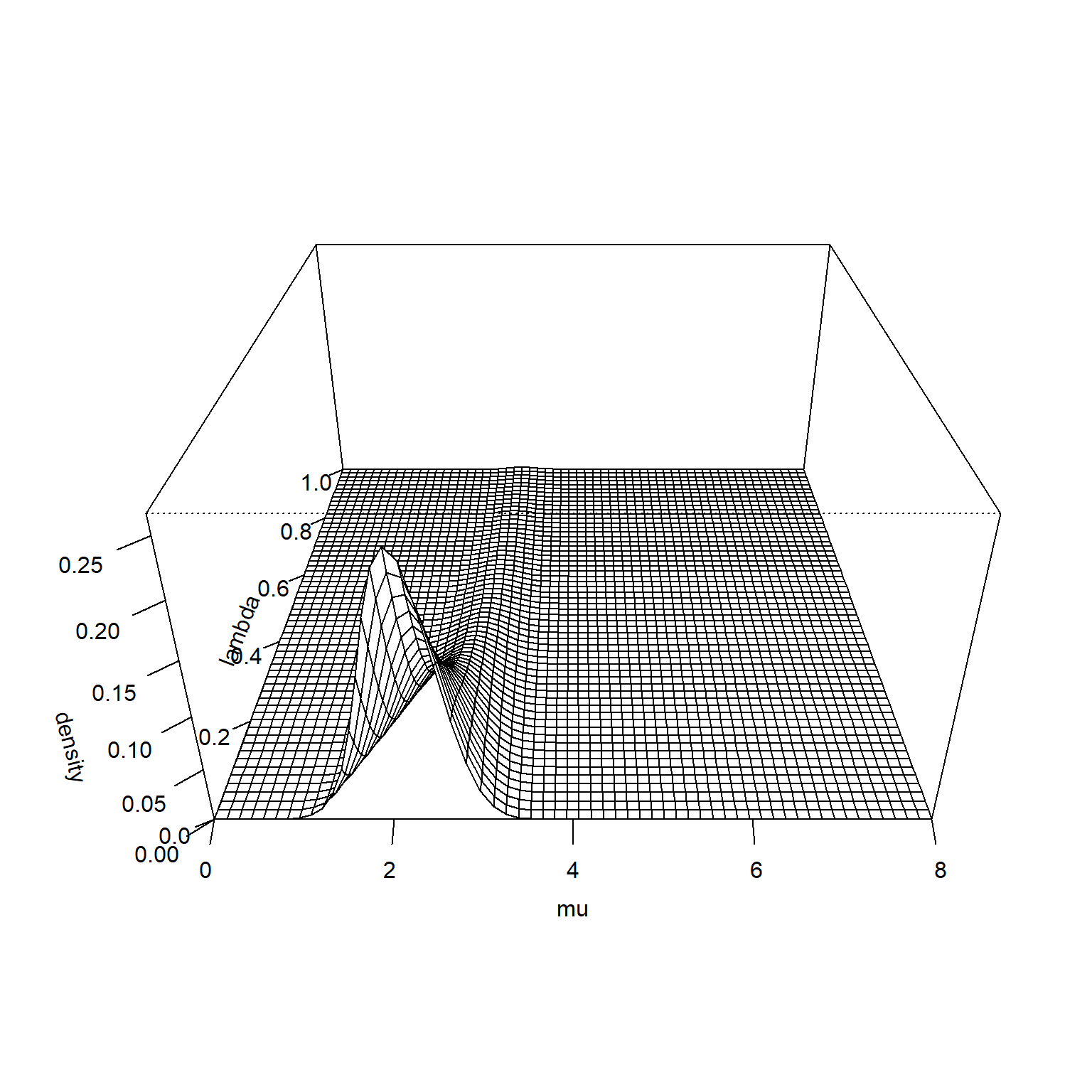A contour plot shows where the probability is concentrated. The labels on the contour lines represent how much probabilty is found inside the contour line.

density <- density / sum(density, na.rm=TRUE)
density %>%
as.vector %>%
sort %>%
rev -> sorted_density
cumulative_p <- 0
for (p in sorted_density) {
cumulative_p <- p + cumulative_p
density[density==p] <- cumulative_p
}
contour(
mu_grid,
lambda_grid,
density,
labcex=1,
levels=c(50, 75, 90)/100,
xlab="mu",
ylab="lambda")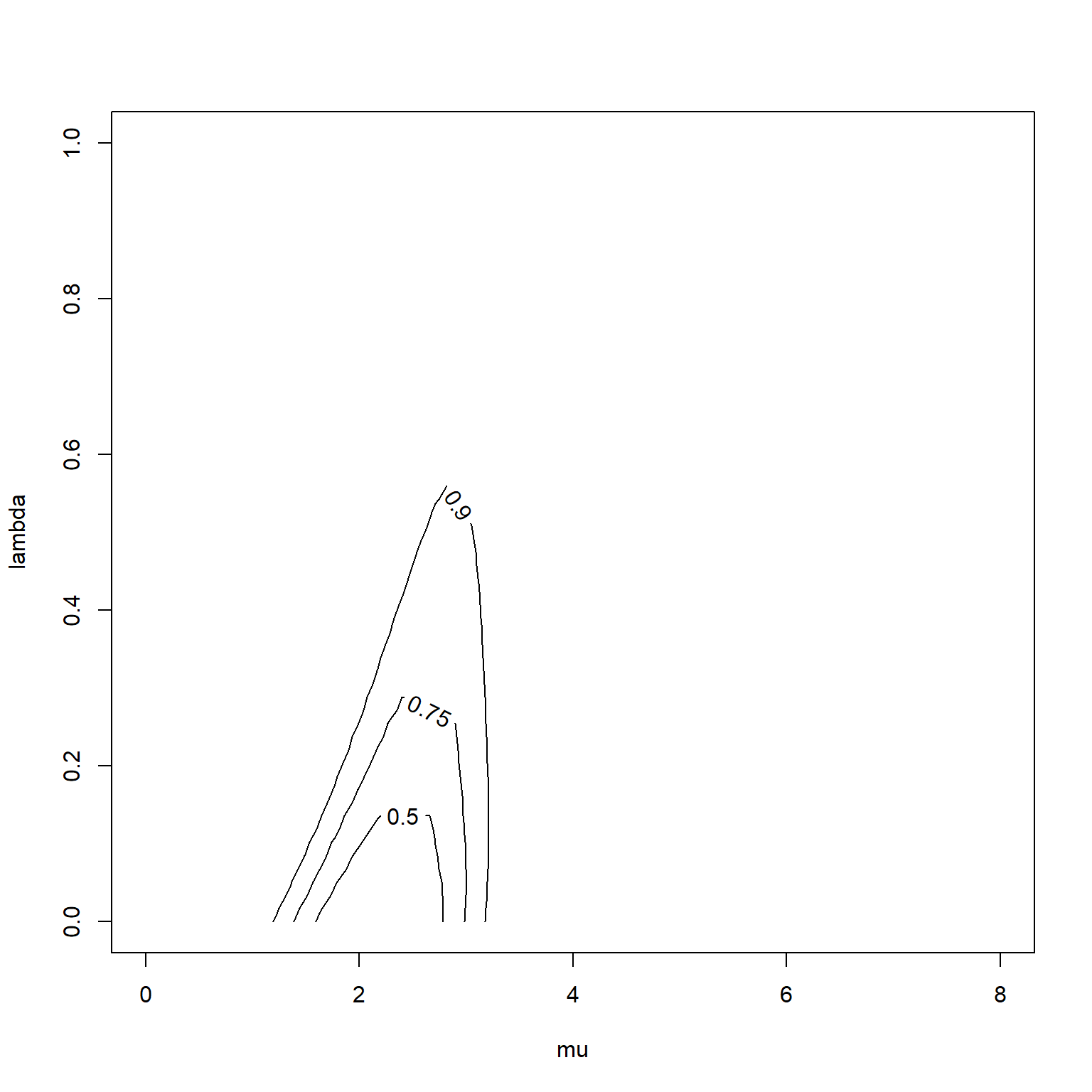You can see that the hedging hyperprior did its job. The most probable values of $$\lambda$$ are small and the prior mean (4) is largely ignored in favor of the mean of the data (2).